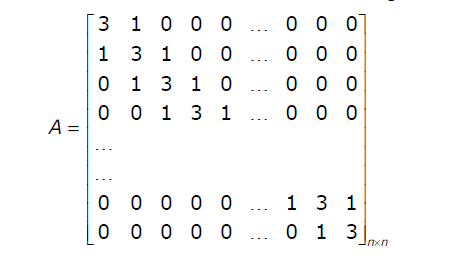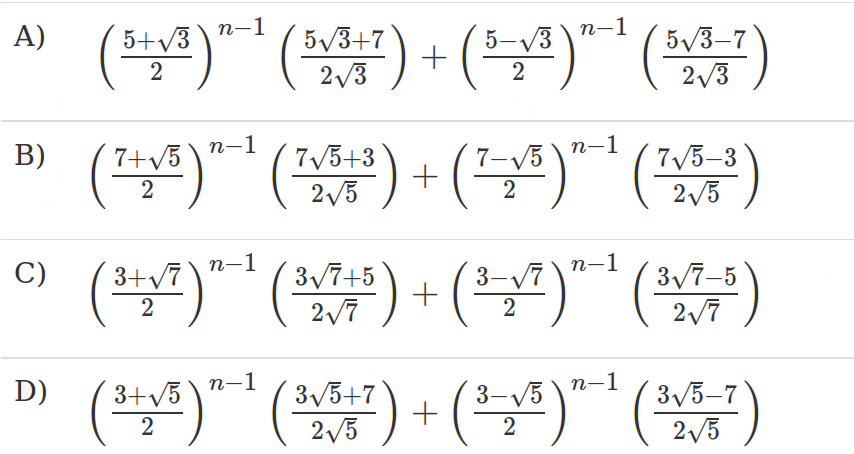# GATE | GATE-IT-2004 | Question 32

• Last Updated : 28 Jun, 2021

Let A be an Let A be an n × n matrix of the following form.What is the value of the determinant of A?(A) A
(B) B
(C) C
(D) D

Explanation:

```The first thing you need to get by seeing these type of questions is:-
Go for substitution method.
For n=2, the values will be
A) 16
B) 26
C) 7
D) 8
As all the values are unique for a small value of n, it does not take much time.
The given matrix will be A = [3 1]
[1 3]
So, det(A) = 3*3-1*1 = 8
```
```   Alternative method:-
You can frame the relations in between det(An+1), det(An), det(An-1)
i.e. d(An+1) = 3*d(An) - d(An-1)
X   = 3*X^0 - X^-1
X^2 = 3*x - 1
Solution for this equation is (3+sqrt(5))/2, (3-sqrt(5))/2
The only option which has roots of type (3+sqrt(5)) is D.
From this, you can match the options easily.
```

This explanation has been provided by Anil Saikrishna.

Quiz of this Question

My Personal Notes arrow_drop_up